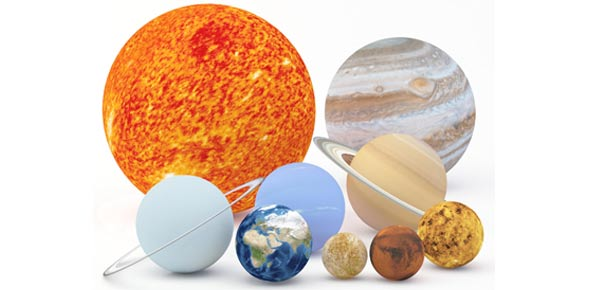# Astronomy Test: Can You Pass?

100 Questions | Total Attempts: 156SettingsAstronomy is a natural science, and it's applies maths, physics, chemistry and geography, Let's start and know more about it!

• 1.
The basic unit of length in the metric system is the
• A.

Micron

• B.

Meter

• C.

Mile

• D.

Kilometer

• 2.
Which of the following numbers has the same meaning as 8670
• A.

8.67 x 10 ^ 3

• B.

8.67 x 10 ^ 2

• C.

8.67 x 10 ^ 1

• D.

0.867 x 10 ^ 3

• 3.
The distance traveled by light or by an object is equal to speed times
• A.

Frequency

• B.

Meters

• C.

Mass

• D.

Time

• 4.
The procedure of writing numbers as a decimal value between 1 and 10 times 10 raised toa n integer power (e.g., 2.4 x 10 ^4) is called
• A.

The scientific method

• B.

Mathematical precision

• C.

Scientific notation

• D.

Astronomical designation

• 5.
A light-year is defined as the
• A.

Speed of light in a vacuum

• B.

Average distance from the Earth to the Sun

• C.

Length of the Solar Year

• D.

Distance light travels in one year

• 6.
Which unit of measure, often used by astronomers, is not a preferred SI unit of combination?
• A.

Meter

• B.

Second

• C.

Kg/m^3

• D.

Km/s

• E.

Angstrom

• 7.
• A.

3 m/s

• B.

300 m/s

• C.

300 km/s

• D.

300,000 km/s

• 8.
The process of breaking light down into its component colors creates a(n)
• A.

Image

• B.

Hologram

• C.

Spectrum

• D.

Pulse

• 9.
Ancient astronomers divided the sky into regions containing distinct groups of stars called
• A.

Clusters

• B.

Coordinates

• C.

Constellations

• D.

Galaxies

• E.

Nebula

• 10.
Scientific results must be
• A.

Hyptothetical

• B.

Reproducible

• C.

Controversial

• D.

Believed by at least 50% of scientists

• 11.
The Earth's atmosphere shields us from most kinds of electromagnetic radiation. The exceptions are
• A.

• B.

• C.

Only visible light frequencies.

• D.

X-rays and gamma rays

• E.

• 12.
Compared with visible-light photons, a photon of radio waves has
• A.

Less energy.

• B.

More energy

• C.

The same energy

• D.

Redder energy

• E.

AM energy.

• 13.
The type of electromagnetic radiation with a wavelength just longer than that of red light is
• A.

• B.

X-rays.

• C.

• D.

Gamma rays.

• E.

• 14.
The rule describing the relationship between the temperature of a material and the wavelength of its peak emittedradiation is
• A.

The blackbody rule.

• B.

The quantum theory.

• C.

Planck's law.

• D.

The Stefan-Boltzmann law.

• E.

Wien's law.

• 15.
The rule describing the relationship between the total radiant energy emitted by a blackbody to its temperature is
• A.

The blackbody rule.

• B.

The quantum theory.

• C.

Planck's law.

• D.

The Stefan-Boltzmann law.

• E.

The Wien displacement law.

• 16.
The atomic model that places electrons in definite orbits around the nucleus but allows only certain orbits to exist isthe
• A.

Bohr model.

• B.

Einstein model.

• C.

Feynman model.

• D.

Rutherford model.

• E.

Thomson model.

• 17.
If electrons are or removed from a neutral atom the atom is said to be
• A.

Nuclear.

• B.

Ionized.

• C.

Doubly neutral.

• D.

Electronic.

• E.

A proto-atom.

• 18.
The lowest possible energy state of an atom is called the
• A.

Excited state.

• B.

Ground state.

• C.

Neutral state.

• D.

Photon level.

• E.

Balmer level.

• 19.
When a gas removes energy at a particular wavelength from radiation passing through it, it creates a(n)
• A.

Continuous spectrum.

• B.

Emission line.

• C.

Stellar spectrum.

• D.

Absorption line.

• E.

Black hole.

• 20.
In a spectrum, bright lines that appear at wavelengths where there is more radiation than at neighboring wavelengthsare called
• A.

Absorption lines.

• B.

Emission lines.

• C.

Fraunhofer lines.

• D.

Continuum lines

• E.

Stellar lines.

• 21.
A curved lens or mirror can form an image by bringing light to a(n)
• A.

Aberration.

• B.

Focus.

• C.

Resolution.

• D.

Divergence.

• E.

Ray.

• 22.
A reflecting telescope that uses a secondary mirror to reflect light to the side of the telescope is called a(n)
• A.

Newtonian.

• B.

Cassegrain.

• C.

Schmidt.

• D.

Gregorian.

• E.

Halleyan.

• 23.
All other things being equal, and ignoring atmospheric effects, a reflecting telescope with a large objective mirror willhave, compared with a telescope with a smaller mirror,
• A.

Better light-gathering power only

• B.

Better resolution only.

• C.

Better light-gathering power and better resolution.

• D.

Better light-gathering power but poorer resolution.

• E.

Better infrared sensitivity.

• 24.
Today, sites for new large ground-based telescopes are selected primarily on the basis of all the following except
• A.

Proximity to universities and research centers

• B.

Elevation above sea level.

• C.

The characteristics of the atmosphere above the proposed site.

• D.

Ability to see the southern sky.

• E.

Dark sky.

• 25.
The Hubble Space Telescope is a(n)
• A.

Ground-based refracting telescope

• B.

Ground-based reflecting telescope.

• C.

Orbiting refracting telescope.

• D.

Orbiting reflecting telescope.

• E.Back to top